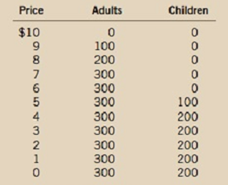Chapter 15, Problem 8PA

Chapter
Section
Textbook Problem

You live in a town with 300 Adults and 200 children, and you arc thinking about putting on a play to entertain your neighbors and make some money. A play has a fixed cost of $2,000, but selling an extra ticket has zero marginal cost. Here are the demand schedules for your two types of customer:a. To maximize profit, what price would you charge for an adult ticket? For a child's ticket? How much profit do you make?b. The city council passes a law prohibiting you from charging different prices to different customers. What price do you set for a ticket now? How much profit do you make?c. Who is worse off because of the law prohibiting price discrimination? Who is better off? (If you can, quantify the changes in welfare.)d. If the fixed cost of the play were$2,500 rather than $2,000, how would your answers to parts (a), (b), and (c) change? Subpart (a): To determine Price discrimination and revenue and profits. Explanation Total revenue can be calculated by using the following formula. Total Revenue=Price × Quantity (1) Substitute the respective values in Equation (1) to calculate the total revenue at 100 for adults. Total revenue=9×100=900 Total revenue is$900.

Since no variable cost is involved, the profit-maximizing outcome is same as the maximizing total revenue. The total revenue obtained from selling to each type of consumer is depicted in Table 1 using Equation (1).

 Price Quantity of Adult Tickets Total Revenue from Sale of Adult Tickets Quantity of Child Tickets Total Revenue from Sale of Child Tickets 10 0 0 0 0 9 100 900 0 0 8 200 </

Subpart (b):

To determine
Price discrimination and revenue and profits.

Subpart (c):

To determine
Price discrimination and revenue and profits.

Subpart (d):

To determine
Price discrimination and revenue and profits.

Still sussing out bartleby?

Check out a sample textbook solution.

See a sample solution

The Solution to Your Study Problems

Bartleby provides explanations to thousands of textbook problems written by our experts, many with advanced degrees!

Get Started